NCERT Exemplar - MCQ

Chapter 7 Class 10 Coordinate Geometry
Serial order wise

## (C) equilateral triangle   (D) scalene triangle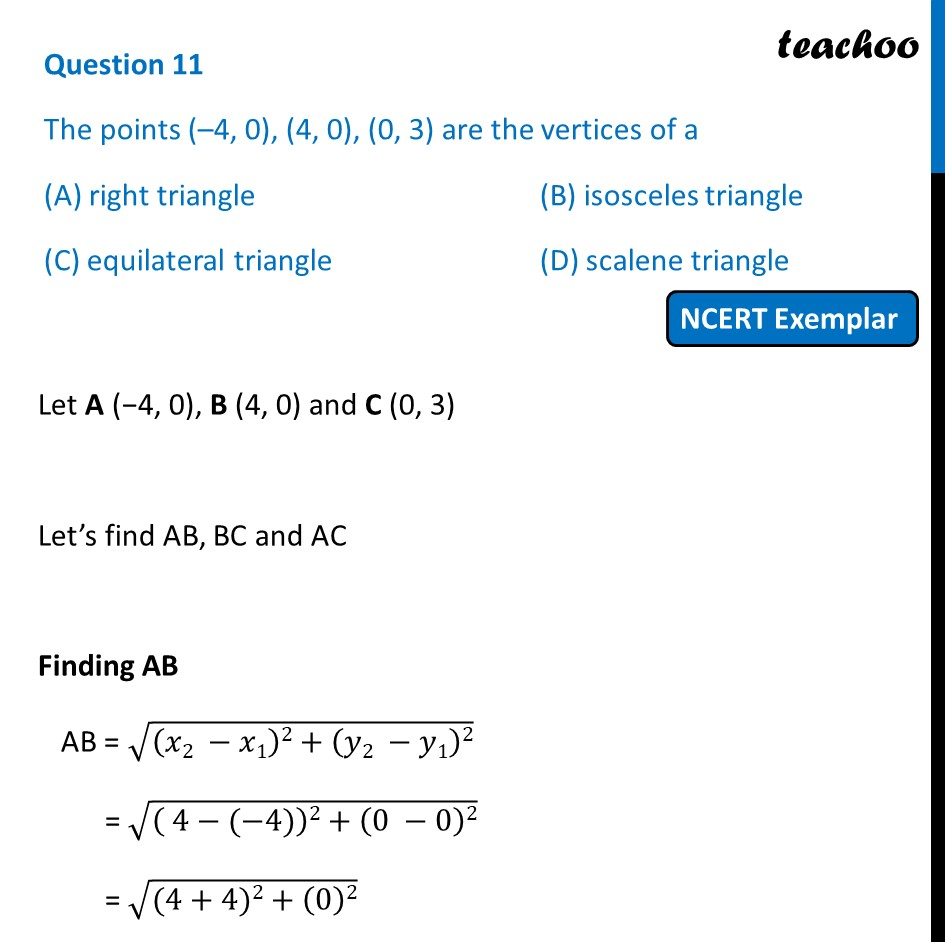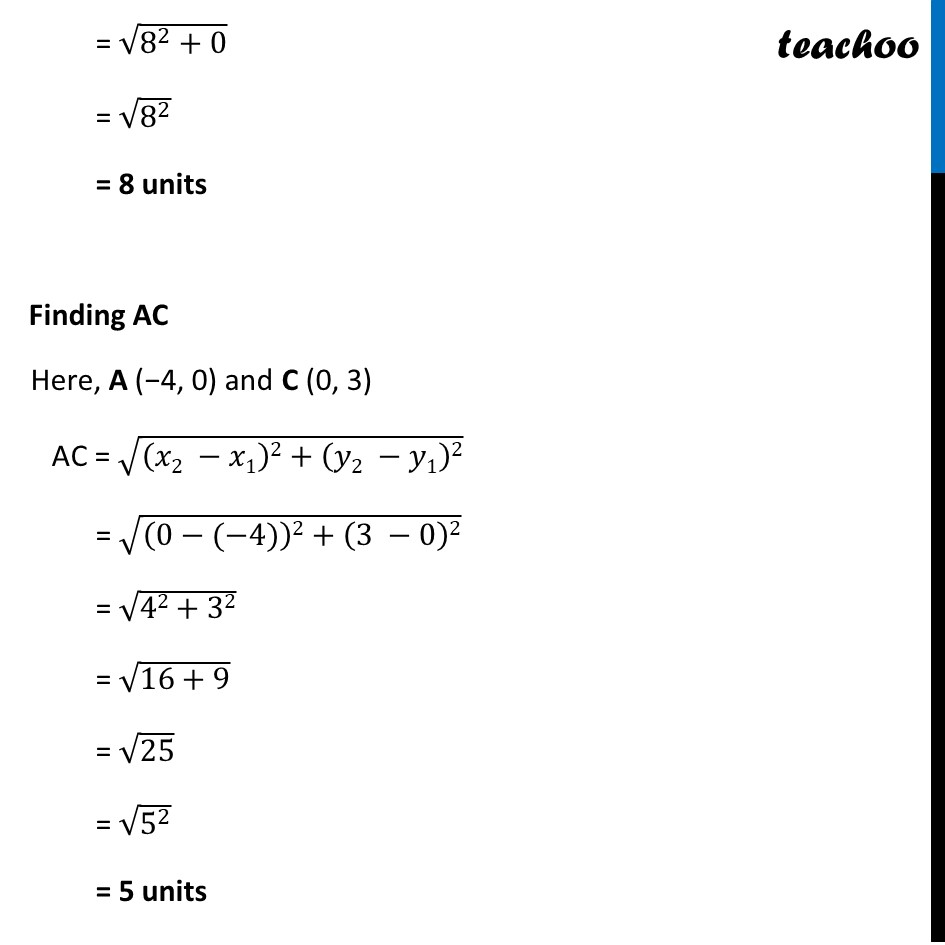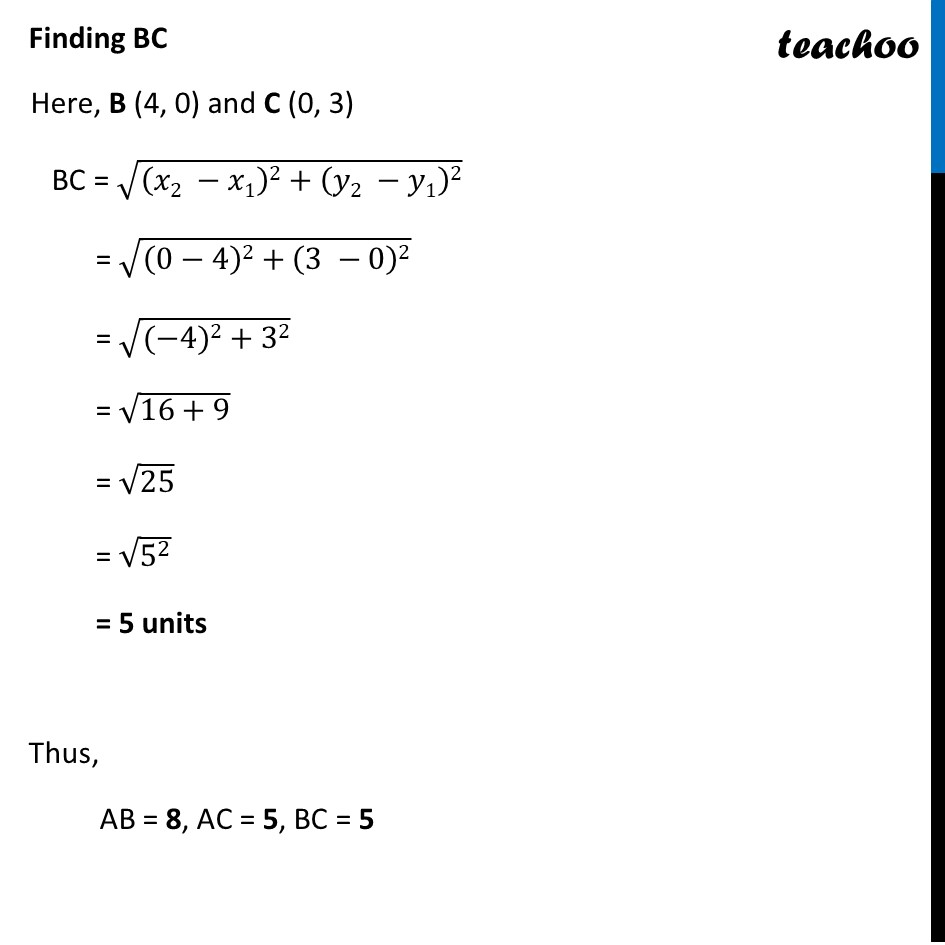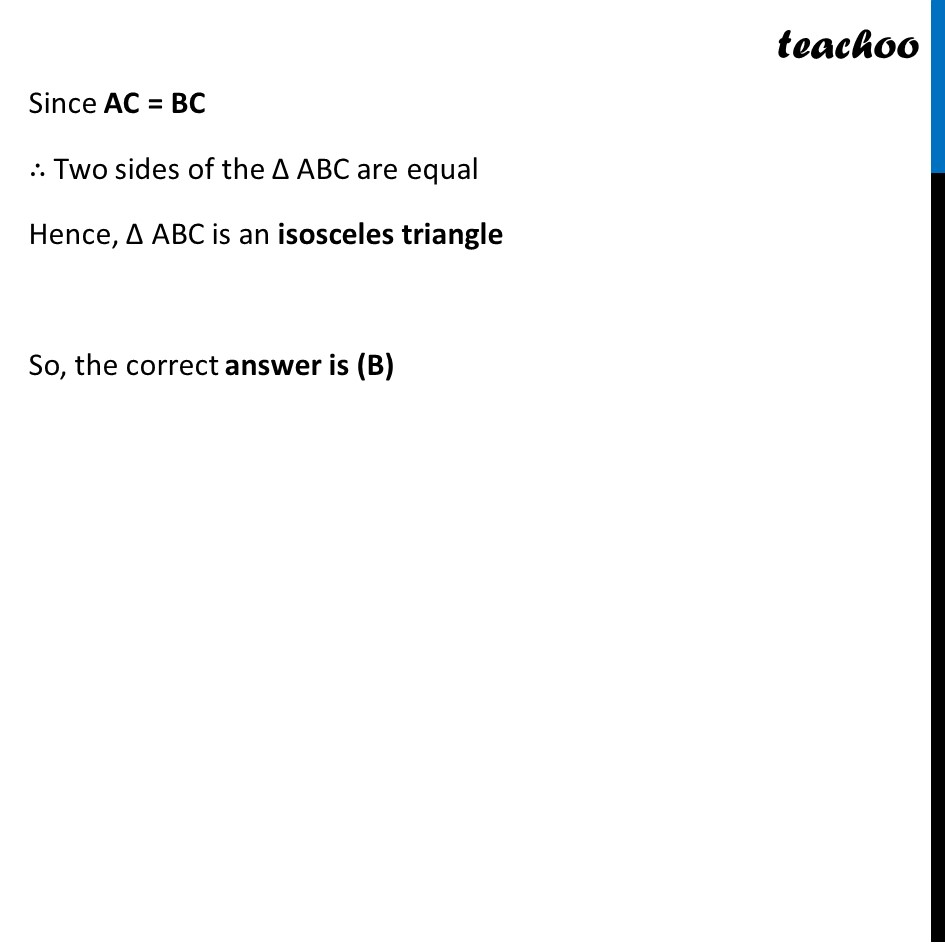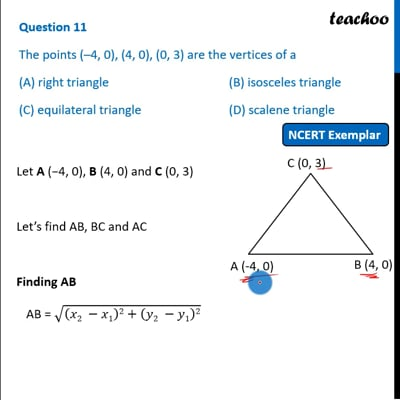This video is only available for Teachoo black users

Introducing your new favourite teacher - Teachoo Black, at only ₹83 per month

### Transcript

Question 11 The points (–4, 0), (4, 0), (0, 3) are the vertices of a (A) right triangle (B) isosceles triangle (C) equilateral triangle (D) scalene triangle Let A (−4, 0), B (4, 0) and C (0, 3) Let’s find AB, BC and AC Finding AB AB = √((𝑥2 −𝑥1)2+(𝑦2 −𝑦1)2) = √(( 4−(−4))2+(0 −0)2) = √((4+4)2+(0)2) = √(8^2+0) = √(8^2 ) = 8 units Finding AC Here, A (−4, 0) and C (0, 3) AC = √((𝑥2 −𝑥1)2+(𝑦2 −𝑦1)2) = √((0−(−4))2+(3 −0)2) = √(42+32) = √(16+9) = √25 = √(5^2 ) = 5 units Finding BC Here, B (4, 0) and C (0, 3) BC = √((𝑥2 −𝑥1)2+(𝑦2 −𝑦1)2) = √((0−4)2+(3 −0)2) = √((−4)2+32) = √(16+9) = √25 = √(5^2 ) = 5 units Thus, AB = 8, AC = 5, BC = 5 Since AC = BC ∴ Two sides of the Δ ABC are equal Hence, Δ ABC is an isosceles triangle So, the correct answer is (B)